Function Repository Resource:

Compute the radial curve of a given curve

Contributed by: Eric W. Weisstein
 ResourceFunction["RadialCurve"][c,p,t] computes the radial curve of the curve c in parameter t with respect to the point p.

## Details and Options

The radial curve is the locus traced by the curvature vector of the given curve, starting at a given point in the plane.

## Examples

### Basic Examples (3)

Obtain the parametric form of an astroid:

 In:=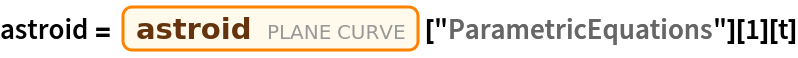Out=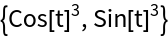Compute the parametric form for the radial curve of the astroid:

 In:=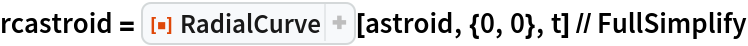Out=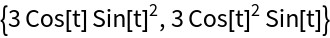Plot the radial curve of the astroid (red):

 In:=Out=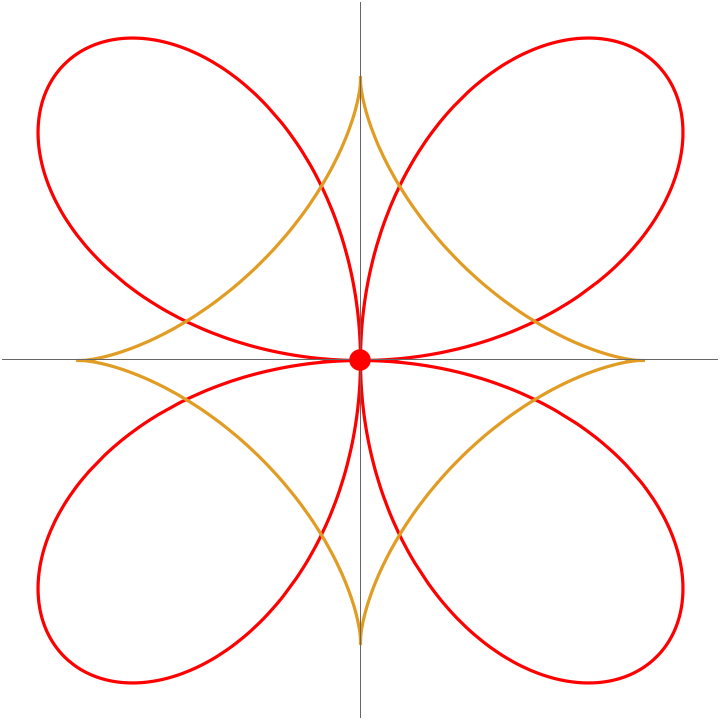### Scope (2)

Define an epicycloid:

 In:=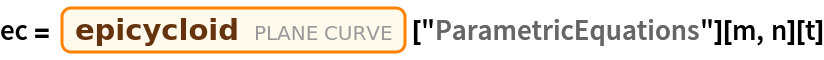Out=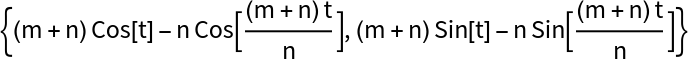A table of plots of epicycloids for different values of m, n:

 In:=Out=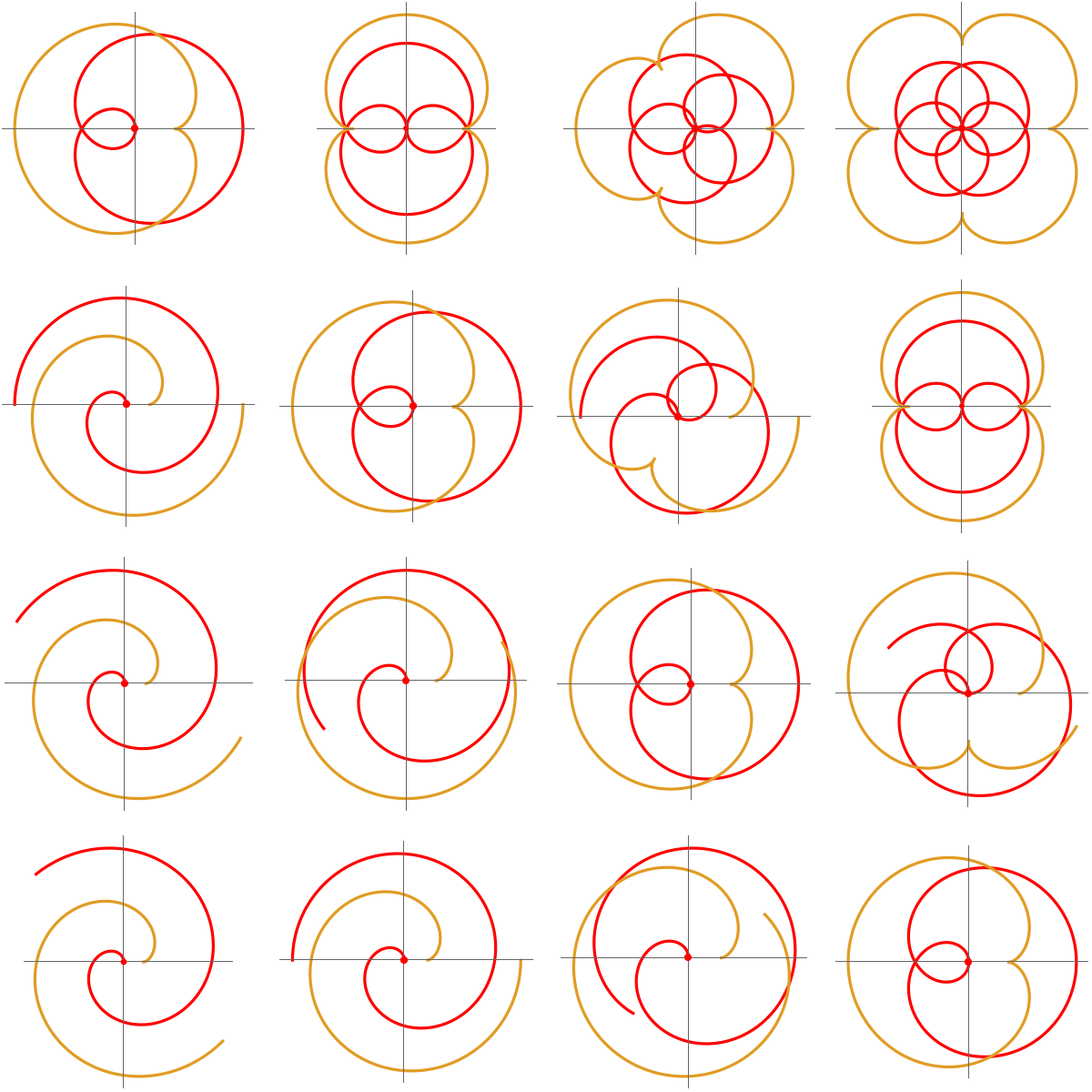Enrique Zeleny

## Version History

• 1.0.0 – 18 March 2020